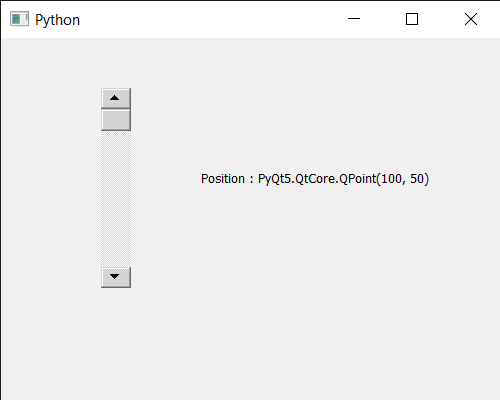Related Articles

# PyQt5 QScrollBar – Getting Its Position in Window

• Last Updated : 31 Jul, 2020

In this article we will see how we can get position of QScrollBar. QScrollBar is a control that enables the user to access parts of a document that is larger than the widget used to display it. Slider is the scroll-able object inside the bar. This property holds the single step. Position is the place where scrool bar is placed in the window. Position can be set with the help of `setGeometry` method.

In order to do this we will use `pos` method with the scroll bar object.

Syntax : scroll.pos()

Argument : It takes no argument

Return : It returns QPoint object

Below is the implementation

 `# importing libraries``from` `PyQt5.QtWidgets ``import` `*` `from` `PyQt5 ``import` `QtCore, QtGui``from` `PyQt5.QtGui ``import` `*` `from` `PyQt5.QtCore ``import` `*` `import` `sys`` ` ` ` `class` `Window(QMainWindow):`` ` `    ``def` `__init__(``self``):``        ``super``().__init__()`` ` `        ``# setting title``        ``self``.setWindowTitle(``"Python "``)`` ` `        ``# setting geometry``        ``self``.setGeometry(``100``, ``100``, ``500``, ``400``)`` ` `        ``# calling method``        ``self``.UiComponents()`` ` `        ``# showing all the widgets``        ``self``.show()`` ` ` ` ` ` `    ``# method for components``    ``def` `UiComponents(``self``):`` ` `        ``scroll ``=` `QScrollBar(``self``)`` ` `        ``# setting geometry of the scroll bar``        ``scroll.setGeometry(``100``, ``50``, ``30``, ``200``)`` ` `        ``# making its background color to green``        ``scroll.setStyleSheet(``"background : lightgrey;"``)`` ` `        ``# creating a label``        ``label ``=` `QLabel(``"GeesforGeeks"``, ``self``)`` ` `        ``# setting geometry to the label``        ``label.setGeometry(``200``, ``100``, ``300``, ``80``)`` ` `        ``# making label multi line``        ``label.setWordWrap(``True``)`` ` `        ``# getting value changed signal``        ``scroll.valueChanged.connect(``lambda``: do_action())`` ` `        ``# method called when signal is emitted``        ``def` `do_action():``             ` `            ``# setting text to the label``            ``label.setText(``"Current Value : "` `+` `str``(scroll.value()))`` ` ` ` `        ``# getting position of scroll bar``        ``value ``=` `scroll.pos()`` ` `        ``# setting text to the label``        ``label.setText(``"Position : "` `+` `str``(value))`` ` ` ` ` ` ` ` `# create pyqt5 app``App ``=` `QApplication(sys.argv)`` ` `# create the instance of our Window``window ``=` `Window()`` ` `# start the app``sys.exit(App.``exec``())`

Output :Attention geek! Strengthen your foundations with the Python Programming Foundation Course and learn the basics.

To begin with, your interview preparations Enhance your Data Structures concepts with the Python DS Course. And to begin with your Machine Learning Journey, join the Machine Learning – Basic Level Course

My Personal Notes arrow_drop_up